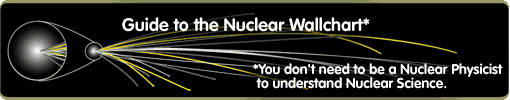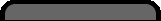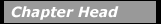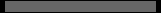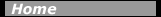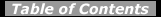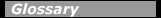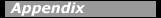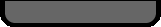## Nuclear Energy LevelsThe nucleus, like the atom, has discrete energy levels whose location and properties are governed by the rules of quantum mechanics. The locations of the excited states differ for each nucleus. The excitation energy, E x, depends on the internal structure of each nucleus. Each excited state is characterized by quantum numbers that describe its angular momentum, parity, and isospin (see chapter 5). Figure 6-1 shows a few of the excited states of the 12C nucleus.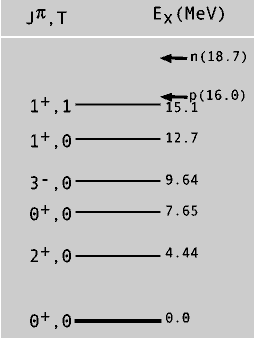Energy level diagram of some of the excited states of the 12C nucleus. The angular momentum (J), parity (P), and isospin (T) quantum numbers of the states are indicated on the left using the notation JP, T. The separation energies for a proton and a neutron are indicated by p and n respectively at the top of the diagram.

The angular momentum quantum number, J, is the integer or half-integer that is the measure of the total angular momentum of the energy state in units of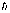(Planck’s constant h divided 2p)

angular momentum = J.

The parity, P, of a nuclear energy level is a statement about what the nuclear structure of the state would look like if the spatial coordinates of all the nucleons were reversed: P = + means the reversed state would look the same as the original; P = - means the reversed state differs from the original. The isospin (projection) quantum number, T, is an integer or half-integer that measures a property that results if neutron and proton coordinates were interchanged. The figure shows these quantum numbers for each excited state in the notation J P, T. These quantum numbers are results of the basic symmetries of the underlying force law that governs the binding of nucleons in a nucleus. They determine how an excited state will decay into another state in the same nucleus (gamma decay) or into a specific state in a different nucleus (beta or alpha decay).

Analyzing the interactions among many nucleons to calculate the energy levels and their properties is a complicated mathematical task. Instead, nuclear scientists have developed several nuclear models that simplified the description the nucleus and the mathematical calculations. These simpler models still preserve the main features of nuclear structure.

#### Chapter Contents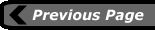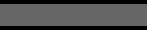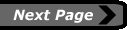last updated: August 9, 2000 webmaster